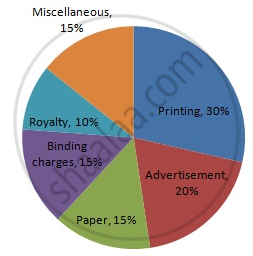Share

# Following Data Gives the Break up of the Cost of Production of a Book: - Mathematics

Course
ConceptCircle Graph Or Pie Chart - Drawing Pie Charts

#### Question

Following data gives the break up of the cost of production of a book:

 Printing Paper Binding charges Advertisement Royalty Miscellaneous 30% 15% 15% 20% 10% 15%

Draw a pie- diagram depicting the above information.

#### Solution

We know:
Central angle of a component = (component value/sum of component values x 360)
Here, total expenditures = 105%
Thus, the central angle for each component can be calculated as follows:

 Item Expenditure(in %) Sector angle Printing 30 30/105 x  360 = 102.9 Paper 15 15/105 x 360 = 51.4 Binding charges 15 15/105 x 360 = 51.4 Advertisement 20 20/105 x 360 = 68.6 Royalty 10 10/105 x 360 = 34.3 Miscellaneous 15 15/105 x 360 = 51.4

Total : 105%
Now, the pie chat representing the given data can be constructed by following the steps below:
Step 1 : Draw circle of an appropriate radius.
Step 2 : Draw a vertical radius of the circle drawn in step 1.
Step 3 : Choose the largest central angle. Here the largest central angle is 102.9o. Draw a sector with the central angle 102.9o in such a way that one of its radii coincides with the radius drawn in step 2 and another radius is in its counter clockwise direction.
Step 4 : Construct other sectors representing the other items in the clockwise sense in descending order of magnitudes of their central angles.
​Step 5 : Shade the sectors with different colours and label them, as shown as in figure below.​​Is there an error in this question or solution?

#### APPEARS IN

RD Sharma Solution for Mathematics for Class 8 by R D Sharma (2019-2020 Session) (2017 to Current)
Chapter 25: Data Handling-III (Pictorial Representation of Data as Pie Charts or Circle Graphs)
Ex. 25.1 | Q: 13 | Page no. 13

#### Video TutorialsVIEW ALL 

Solution Following Data Gives the Break up of the Cost of Production of a Book: Concept: Circle Graph Or Pie Chart - Drawing Pie Charts.
S